# Super Coin Puzzle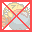Super Coin Puzzle can also be played on mobile devices.Scan the QR code.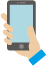## Rules

• Stack adjacent coins of the same amount.
• When the total amount of the stacked coins is the same as the coin more worth, the coins will be exchanged.
*If the total amount exceeds the amount of the coin more worth, the coins will not be exchanged.
•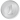x 5 =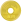•x 2 =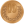•x 5 =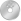•x 2 =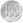•x 5 =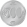• When you exchange to a 500 yen coin, it will go into the piggy bank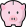automatically.
• The game is cleared when the specified number of 500 yen coins are put in the piggy bank.
• To buy(remove) a sweet, merge it and the coins which is equal to or more worth than its price.
• The game is over when any coins cannot be moved or time is up.
Similar Games